# OECD Household disposable income data analysis 7 - Bootstrap using RPhoto by Toni Lluch on Unsplash

This post is following of above post.
In this post I will do Bootstrap and make confidence inerval of regression.

First, let's check coefficients for static regression model again.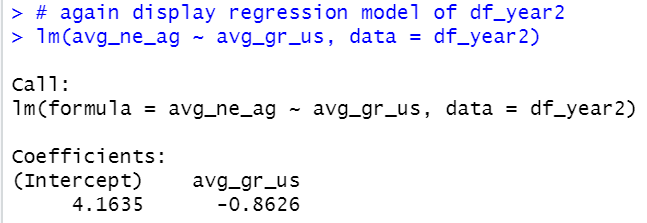Intercept is 4.1635, avg_gr_us is -0.8626.

Then, I make a custom function to get intercept.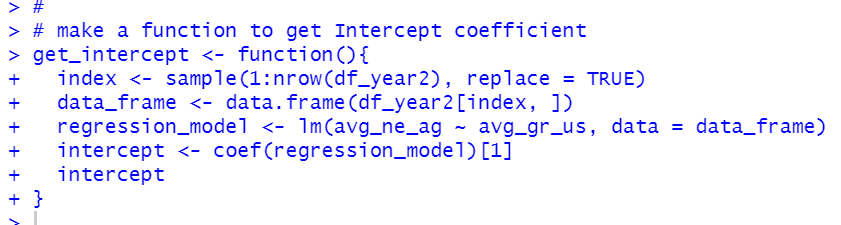Next, I replicate 10,000 times to get intercept.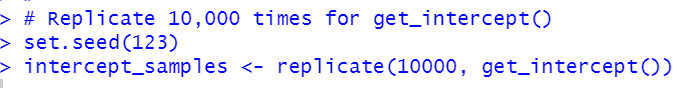Let's see a histogram of intercept_samples.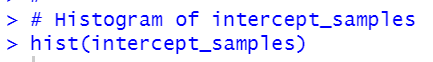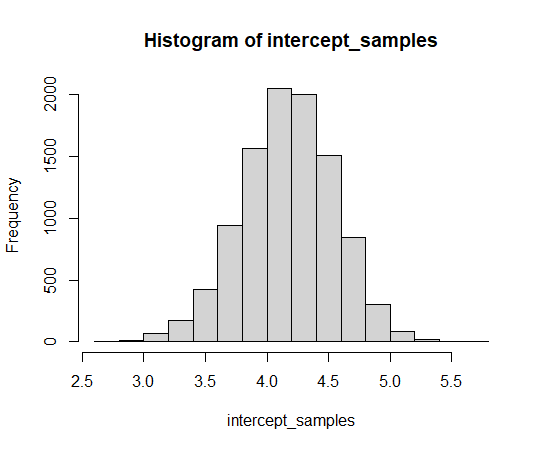Now, we can get 95% confidence interval and mean.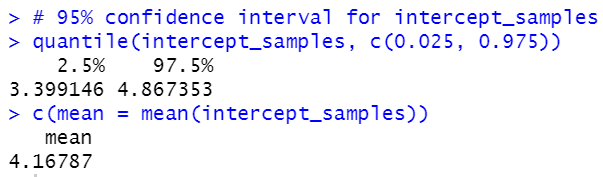Our regression model's intercept is 4.1635. So, bootstrap gets very close intercept value.

We can do the same procedure about slope.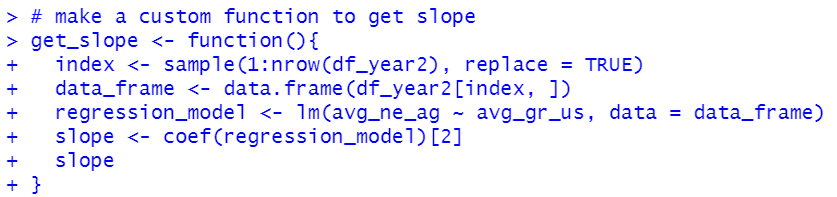I replicate 10,000 times for get_slope().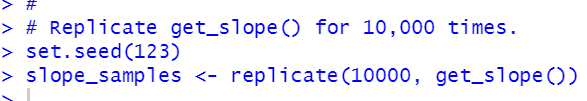Let's see a histogram.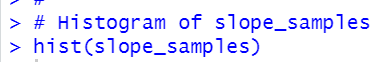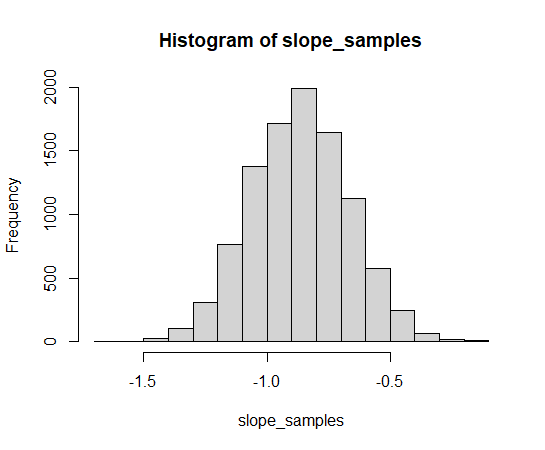Now, I can get 95% confidence interval and mean value.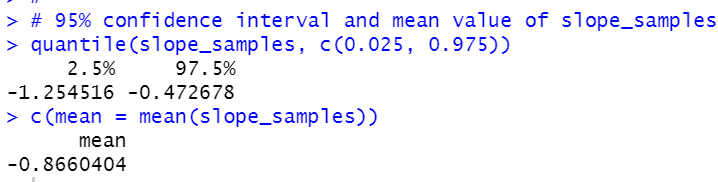Our regression model's slope is -0.8626. So, bootstrap slope is very close to it.

That's it. Thank you!